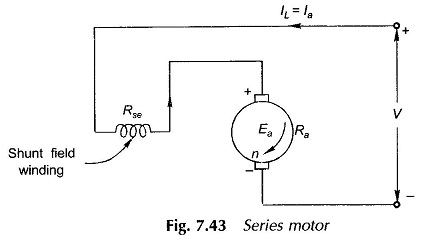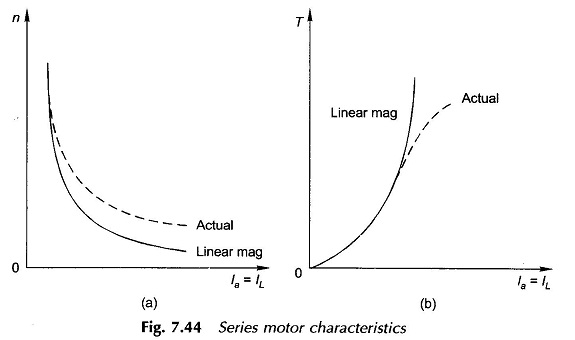## Series Motor

Figure 7.43 shows the connection diagram of a dc series motor. The speed for this motor is (refer to Eq. (7.48)).These relationships are plotted in Figs 7.44(a) and (b). Saturation and armature reaction demagnetization both cause the flux/pole to increase (with respect to /a) at a rate slower than in the assumed linear relationship. Actual characteristics are plotted in dotted lines in Fig. 7.44(a) and (b).It is easily observed that at no-load, the motor current and hence the flux/pole tends to zero and as a consequence (Eq. (7.58)), the motor speed tends to increase to